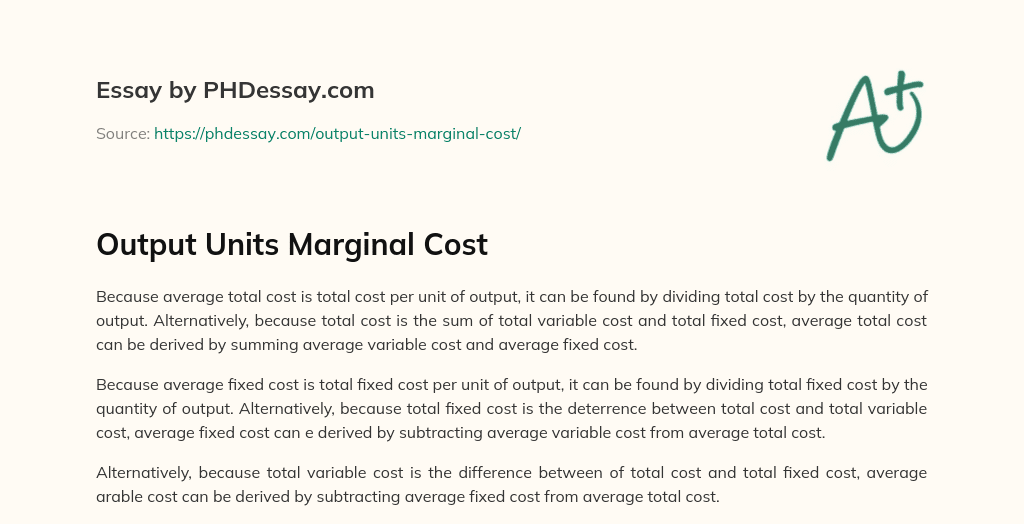Last Updated 07 Jul 2021

# Output Units Marginal Cost

Words 138 (1 page)
25 views

Because average total cost is total cost per unit of output, it can be found by dividing total cost by the quantity of output. Alternatively, because total cost is the sum of total variable cost and total fixed cost, average total cost can be derived by summing average variable cost and average fixed cost.

Because average fixed cost is total fixed cost per unit of output, it can be found by dividing total fixed cost by the quantity of output. Alternatively, because total fixed cost is the deterrence between total cost and total variable cost, average fixed cost can e derived by subtracting average variable cost from average total cost.

Alternatively, because total variable cost is the difference between of total cost and total fixed cost, average arable cost can be derived by subtracting average fixed cost from average total cost.

Order custom essay Output Units Marginal Cost with free plagiarism report

GET ORIGINAL PAPERThis essay was written by a fellow student. You can use it as an example when writing your own essay or use it as a source, but you need cite it.

## Get professional help and free up your time for more important courses

Starting from 3 hours delivery 450+ experts on 30 subjects
get essay help 124  experts online

Did you know that we have over 70,000 essays on 3,000 topics in our database?

Explore how the human body functions as one unit in harmony in order to life

Output Units Marginal Cost. (2018, Jul 12). Retrieved from https://phdessay.com/output-units-marginal-cost/